# 2 2 Linear Equations Linear Equations Linear Function

• Slides: 31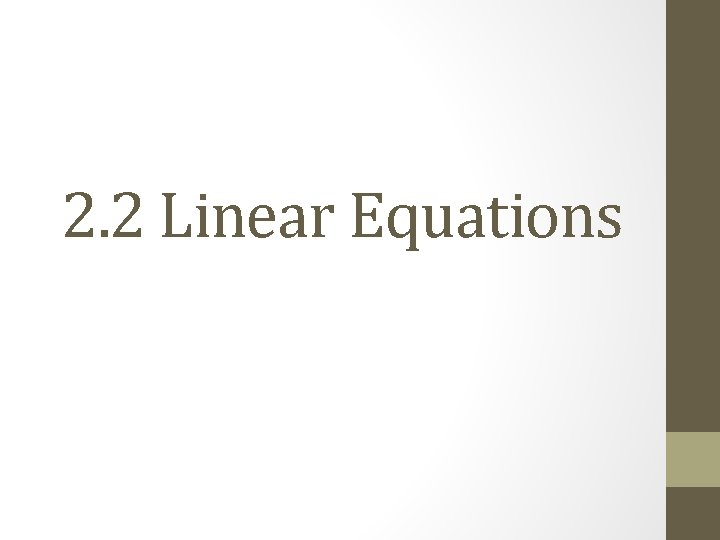2. 2 Linear Equations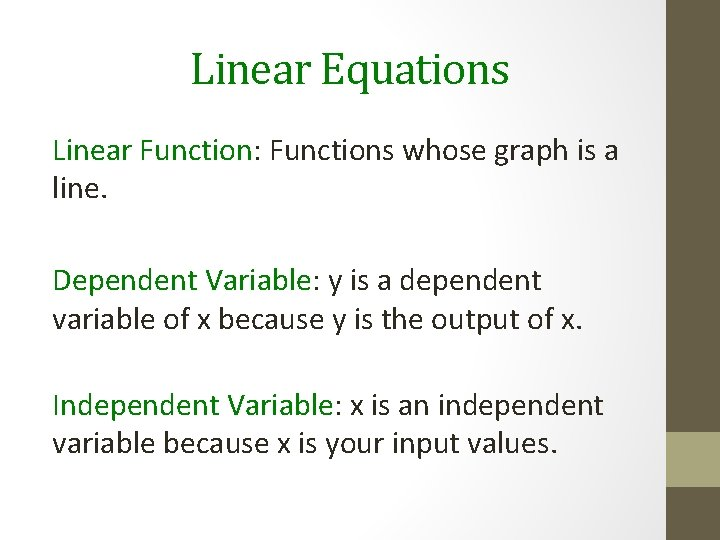Linear Equations Linear Function: Functions whose graph is a line. Dependent Variable: y is a dependent variable of x because y is the output of x. Independent Variable: x is an independent variable because x is your input values.Slope Dude http: //www. teachertube. com/view. Video. php? title=Slope_Dude &video_id=125151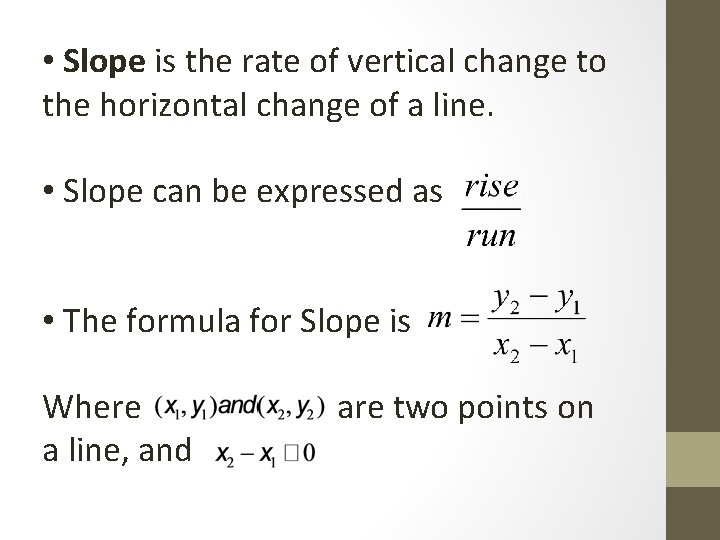• Slope is the rate of vertical change to the horizontal change of a line. • Slope can be expressed as • The formula for Slope is Where a line, and are two points onWhen finding slope from a graph use Pick two points on the line. Starting with the left most point, count rise and run When counting rise: Up is positive rise Down is negative rise When counting run: Right is positive run Left is negative run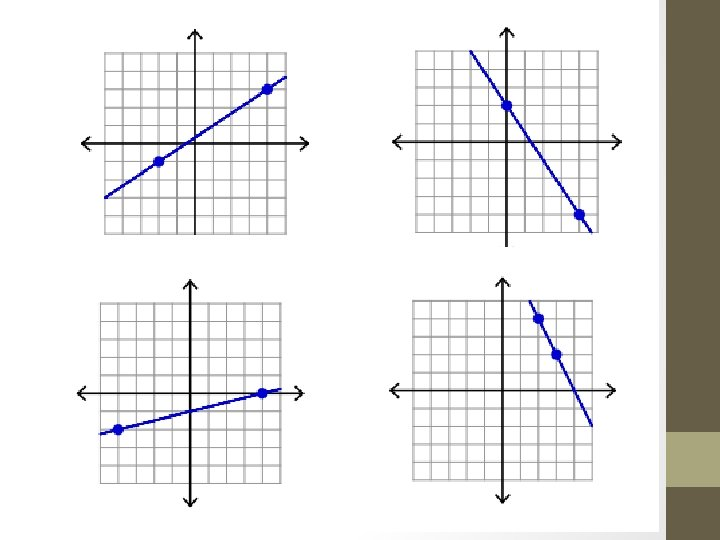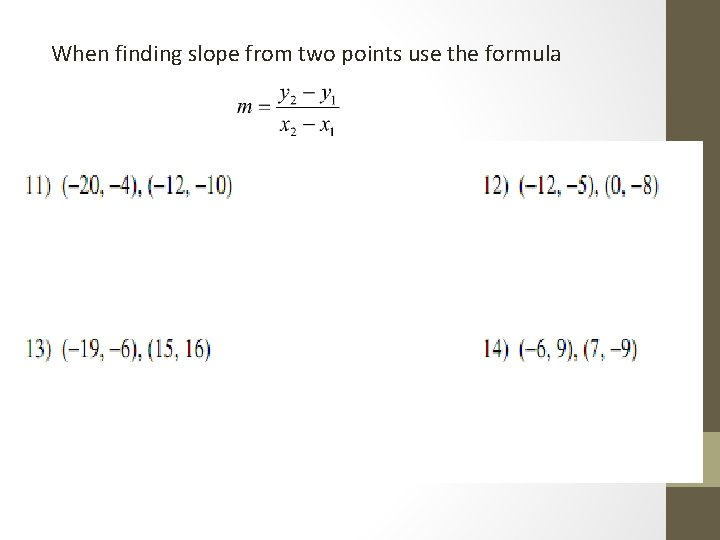When finding slope from two points use the formulaSpecial Cases in Slope Vertical Lines: lines with zero change is the x values have an UNDEFINED slope Horizontal Lines: lines with zero change in the y values and have a ZERO slopeslope-intercept form: • x and y are our variables • m is our slope • b is our y-intercept or where the line crosses the yaxis y-intercept RUN R I S E Slope1) y = (-1/4)x - 2 2) y = x 3) y = (3/4)x + 1 4) y = -2 x + 4Standard Form: Where A, B, and C are coefficients, and x and y are our variables. y-intercept (C/B) is our y-intercept (C/A) is our x-intercept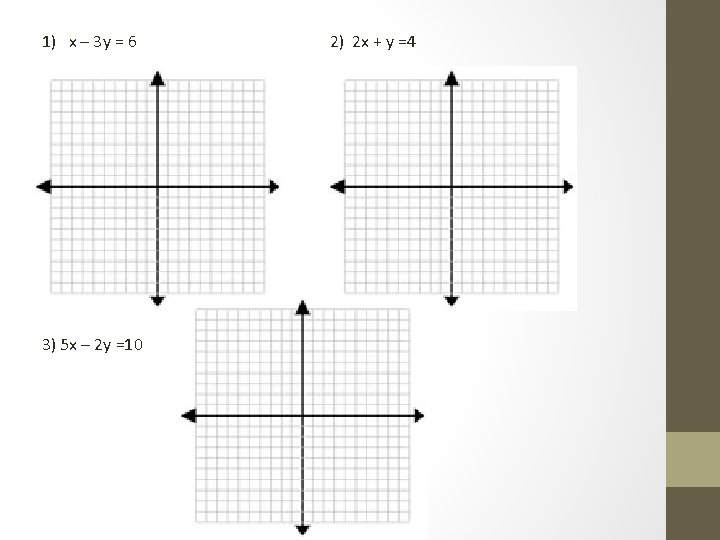1) x – 3 y = 6 3) 5 x – 2 y =10 2) 2 x + y =41) x – 2 y = -4 2) x + 2 y = 0 3) 2 x + 3 y = 6 4) 2 x + y = 1Point-Slope Form: Another form of a linear equation is the point-slope form. This form is mainly used for when the yintercept is not clearly shown on the graph • Where x and y are our variables • m is the slope • And is any point on the line.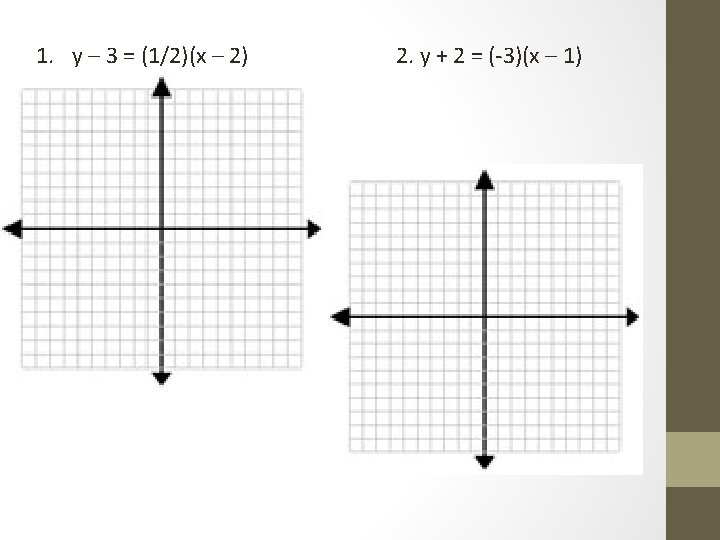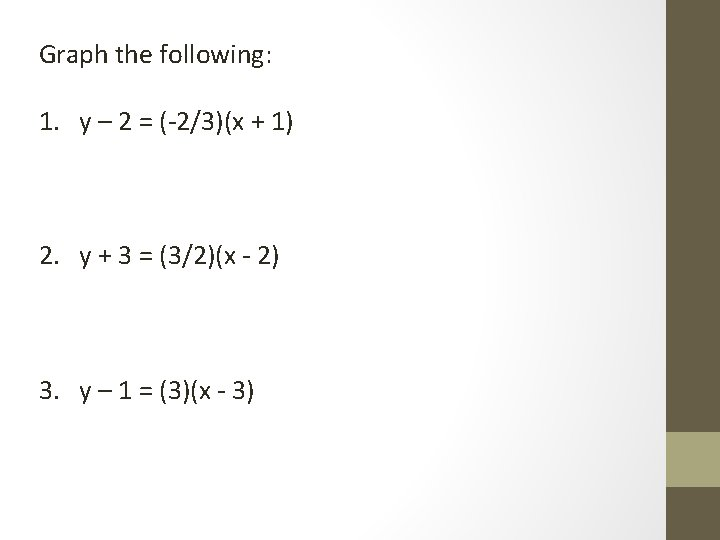Graph the following: 1. y – 2 = (-2/3)(x + 1) 2. y + 3 = (3/2)(x - 2) 3. y – 1 = (3)(x - 3)Writing Linear Equationsslope-Intercept Form: • Y and X are our variables • m = slope • b is our y-intercept, or where the line crosses the y-axisFind the Equation of the line. X Y 0 2 -1 -1 -2 -4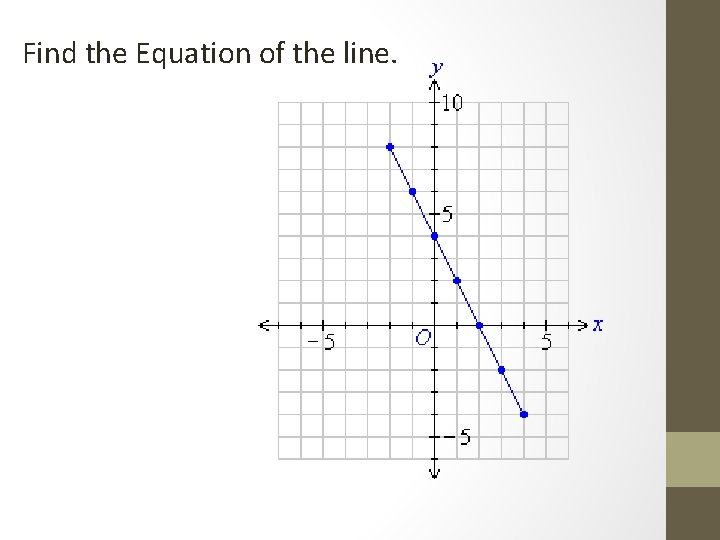Find the Equation of the line.Find the Equation of the line: A line with slope (4/3) that passes through the point (-3, 1).You Try! Find the Equation of the line: A line with an x-intercept of -3 that passes through the point (1, 4).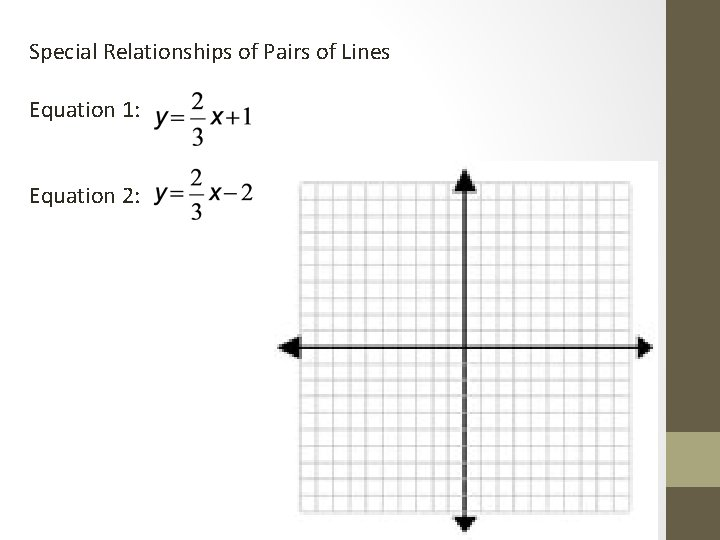Special Relationships of Pairs of Lines Equation 1: Equation 2: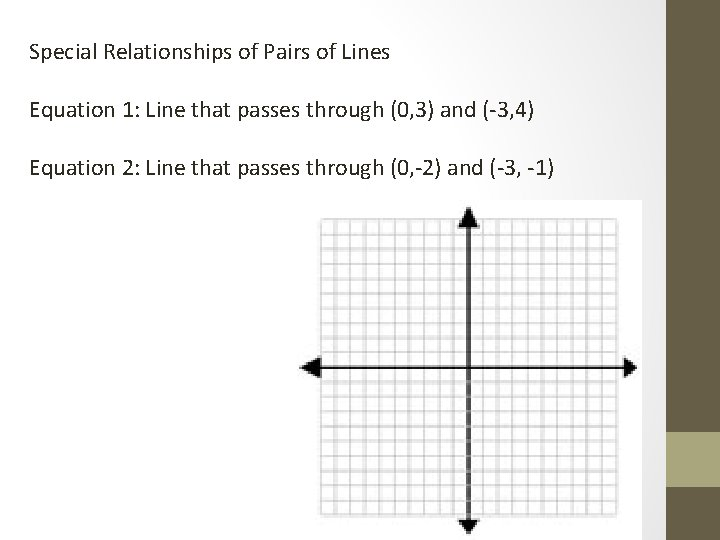Special Relationships of Pairs of Lines Equation 1: Line that passes through (0, 3) and (-3, 4) Equation 2: Line that passes through (0, -2) and (-3, -1)Two lines are parallel when they have the same slope. Parallel lines are coplanar lines that do not intersect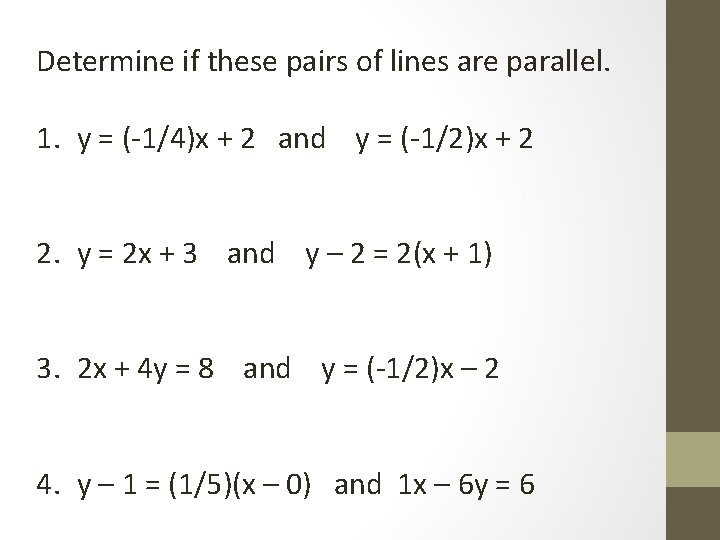Determine if these pairs of lines are parallel. 1. y = (-1/4)x + 2 and y = (-1/2)x + 2 2. y = 2 x + 3 and y – 2 = 2(x + 1) 3. 2 x + 4 y = 8 and y = (-1/2)x – 2 4. y – 1 = (1/5)(x – 0) and 1 x – 6 y = 6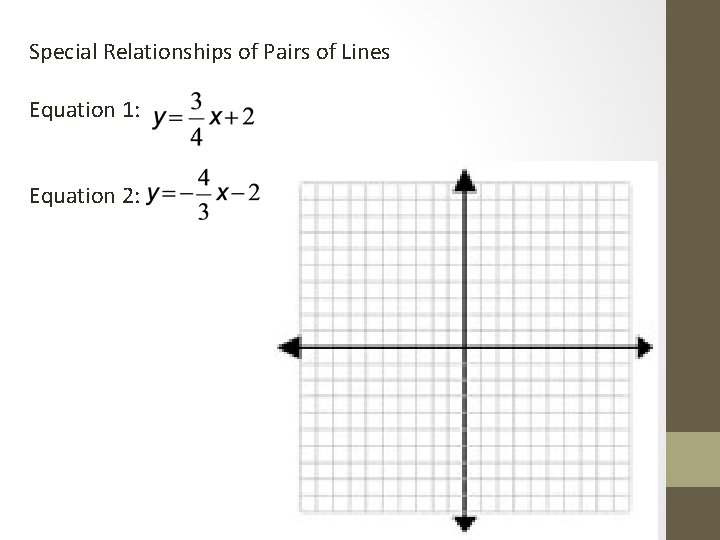Special Relationships of Pairs of Lines Equation 1: Equation 2: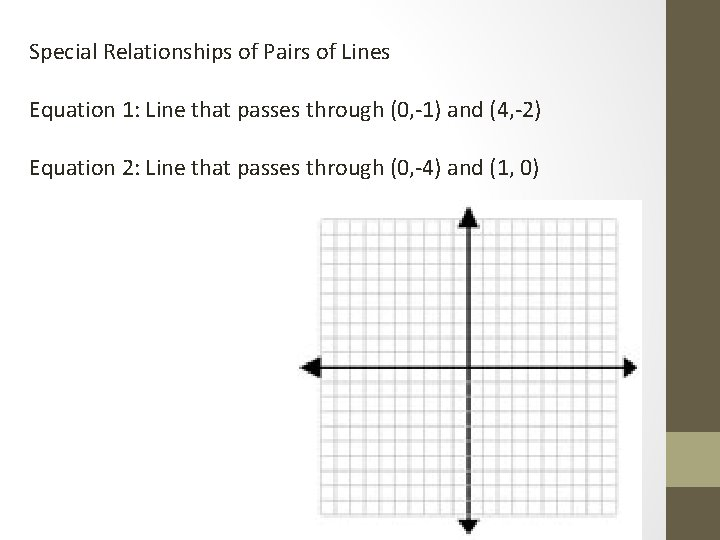Special Relationships of Pairs of Lines Equation 1: Line that passes through (0, -1) and (4, -2) Equation 2: Line that passes through (0, -4) and (1, 0)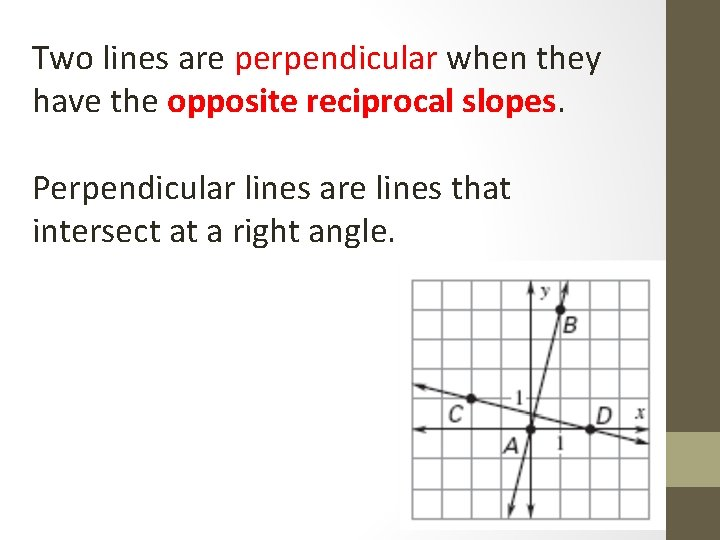Two lines are perpendicular when they have the opposite reciprocal slopes. Perpendicular lines are lines that intersect at a right angle.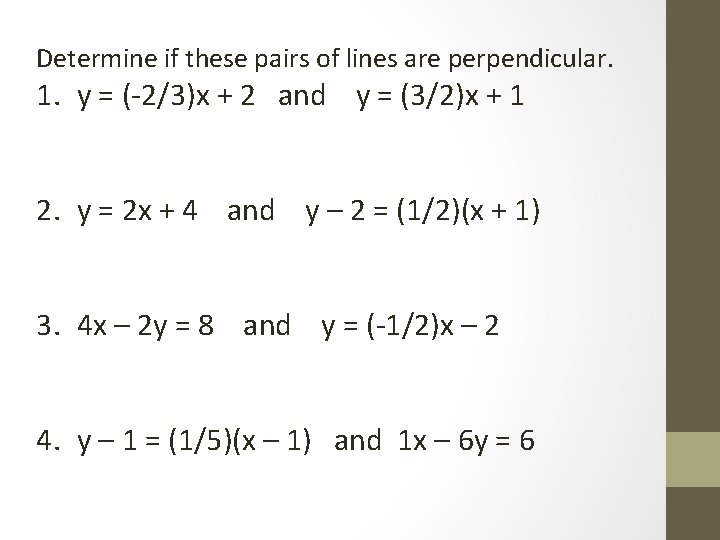Determine if these pairs of lines are perpendicular. 1. y = (-2/3)x + 2 and y = (3/2)x + 1 2. y = 2 x + 4 and y – 2 = (1/2)(x + 1) 3. 4 x – 2 y = 8 and y = (-1/2)x – 2 4. y – 1 = (1/5)(x – 1) and 1 x – 6 y = 6